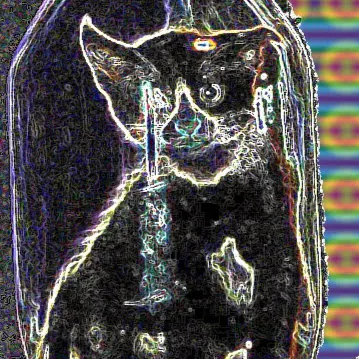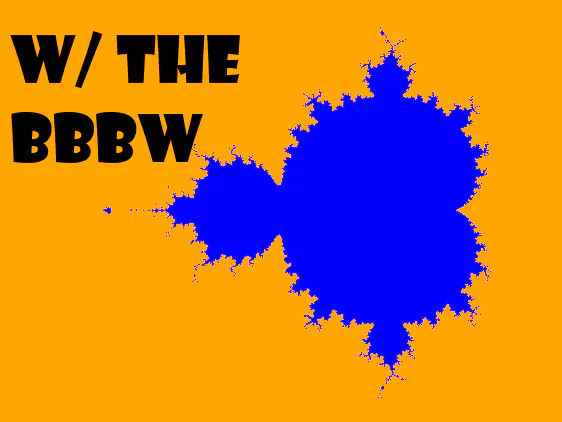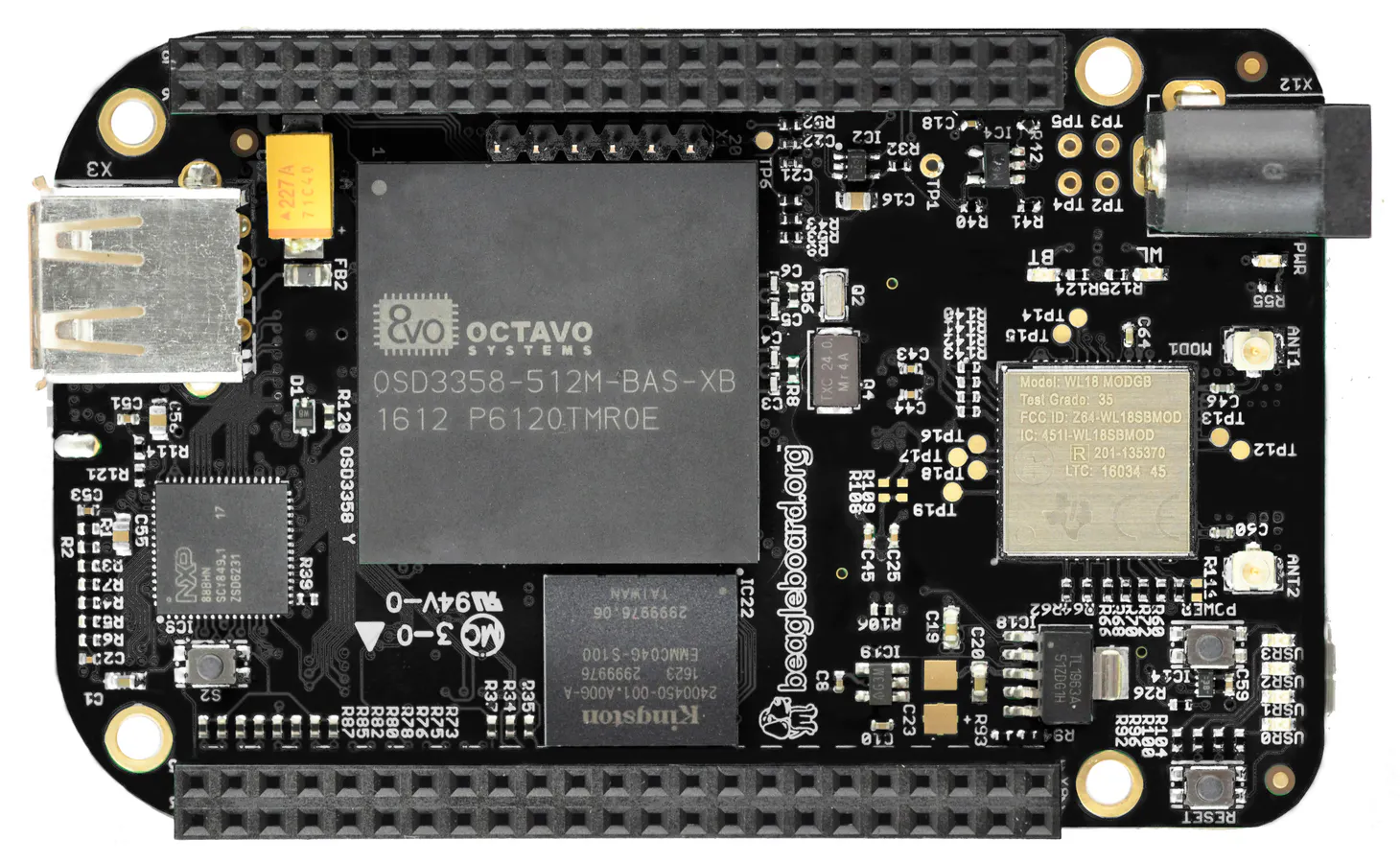Published

# RE: Fractal Imagery From a Python Script on a BBBW

Did you ever want to view a nice visual of a fractal image on an embedded computer with the correct source?

IntermediateProtip1 hour250## Things used in this project

### Hardware componentsBeagleBoard.org BeagleBone Black Wireless
×1

### Software apps and online services

 Python3
 BeagleBoard.org Debian Image

## Code

### Hello

Python
This is the source to run to call your other libraries for producing a fractal image.
```# viewer1.py

from mandel1a import mandel
from viewer import Viewer
import time

import sys
if sys.version_info &lt; (3,):
import Tkinter as tk
range = xrange
else:
import tkinter as tk

class FancyViewer(Viewer):
'''Application to display fractals'''

def draw_pixel(self, x, y):
'''Simulates drawing a given pixel in black by drawing a black line
of length equal to one pixel.'''
self.canvas.create_line(x, y, x+1, y, fill="black") # can be changed

def draw_fractal(self):
'''draws a fractal on the canvas'''
self.calculating = True
begin = time.time()
# clear the canvas
self.canvas.create_rectangle(0, 0, self.canvas_width,
self.canvas_height, fill="white") # can be                                                                       changed
for x in range(0, self.canvas_width):
real = self.min_x + x*self.pixel_size
for y in range(0, self.canvas_height):
imag = self.min_y + y*self.pixel_size
c = complex(real, imag)
if mandel(c, self.nb_iterations):
self.draw_pixel(x, self.canvas_height - y)
self.status.config(text="Time required = %.2f s  [%s iterations]  %s" %(
(time.time() - begin), self.nb_iterations,
self.zoom_info))
self.status2.config(text=self.info())
self.calculating = False

if __name__ == "__main__":
root = tk.Tk()
app = FancyViewer(root)
root.mainloop()
```

### HelloI

Python
```# mandel1a.py

def mandel(c, max_iterations=20):
'''determines if a point is in the Mandelbrot set based on deciding if,
after a maximum allowed number of iterations, the absolute value of
the resulting number is greater or equal to 2.'''
z = 0
for iter in range(0, max_iterations):
z = z**2 + c
if abs(z) >= 2:
return False
return abs(z) < 2
```

### HelloII

Python
```''' viewer.py

Base class viewer for fractals.'''

import sys
if sys.version_info < (3,):
import Tkinter as tk
import tkSimpleDialog as tk_dialog
else:
import tkinter as tk
from tkinter import simpledialog as tk_dialog

class Viewer(object):
'''Base class viewer to display fractals'''

def __init__(self, parent, width=600, height=480,
min_x=-2.5, min_y=-1.5, max_x=1.):

self.parent = parent
self.canvas_width = width
self.canvas_height = height

# The following are drawing boundaries in the complex plane
self.min_x = min_x
self.min_y = min_y
self.max_x = max_x
self.calculate_pixel_size()
self.max_y = self.min_y + self.canvas_height*self.pixel_size

self.calculating = False
self.nb_iterations = 20
self.normal_zoom(None)

self.canvas = tk.Canvas(parent, width=width, height=height)
self.canvas.pack()
self.status = tk.Label(self.parent, text="", bd=1, relief=tk.SUNKEN,
anchor=tk.W)
self.status.pack(side=tk.BOTTOM, fill=tk.X)
self.status2 = tk.Label(self.parent, text=self.info(), bd=1,
relief=tk.SUNKEN, anchor=tk.W)
self.status2.pack(side=tk.BOTTOM, fill=tk.X)

# We change the size of the image using the keyboard.
self.parent.bind("+", self.zoom_in)
self.parent.bind("-", self.zoom_out)
self.parent.bind("n", self.normal_zoom)
self.parent.bind("b", self.bigger_zoom)

# Set the maximum number of iterations via a keyboard-triggered event
self.parent.bind("i", self.set_max_iter)

# We move the canvas using the mouse.
self.translation_line = None
self.parent.bind("button-1 ", self.mouse_down) # edited
self.parent.bind("button1-motion ", self.mouse_motion) # edited
self.parent.bind("button1-buttonrelease ", self.mouse_up) # edited

self.draw_fractal()  # Needs to be implemented by subclass

def info(self):
return "Location: (%f, %f) to (%f, %f)" %(self.min_x, self.min_y,
self.max_x, self.max_y)

def calculate_pixel_size(self):
'''Calculates the size of a (square) pixel in complex plane
coordinates based on the canvas_width.'''
self.pixel_size = 1.*(self.max_x - self.min_x)/self.canvas_width
return

def mouse_down(self, event):
'''records the x and y positions of the mouse when the left button
is clicked.'''
self.start_x = self.canvas.canvasx(event.x)
self.start_y = self.canvas.canvasy(event.y)

def mouse_motion(self, event):
'''keep track of the mouse motion by drawing a line from its
starting point to the current point.'''
x = self.canvas.canvasx(event.x)
y = self.canvas.canvasy(event.y)

if (self.start_x != event.x)  and (self.start_y != event.y) :
self.canvas.delete(self.translation_line)
self.translation_line = self.canvas.create_line(
self.start_x, self.start_y, x, y, fill="orange")

def mouse_up(self, event):
'''Moves the canvas based on the mouse motion'''
dx = (self.start_x - event.x)*self.pixel_size
dy = (self.start_y - event.y)*self.pixel_size
self.min_x += dx
self.max_x += dx
# y-coordinate in complex plane run in opposite direction from
# screen coordinates
self.min_y -= dy
self.max_y -= dy
self.canvas.delete(self.translation_line)
self.status2.config(text=self.info())
self.draw_fractal()

def normal_zoom(self, event, scale=1):
'''Sets the zooming in/out scale to its normal value'''
if scale==1:
self.zoom_info = "[normal zoom]"
else:
self.zoom_info = "[faster zoom]"
if event is not None:
self.status.config(text=self.zoom_info)
self.zoom_in_scale = 0.1
self.zoom_out_scale = -0.125

def bigger_zoom(self, event):
'''Increases the zooming in/out scale from its normal value'''
self.normal_zoom(event, scale=3)
self.zoom_in_scale = 0.3
self.zoom_out_scale = -0.4

def zoom_in(self, event):
'''decreases the size of the region of the complex plane displayed'''
if self.calculating:
return
self.change_scale(self.zoom_in_scale)

def zoom_out(self, event):
'''increases the size of the region of the complex plane displayed'''
if self.calculating:
return
self.change_scale(self.zoom_out_scale)

def change_scale(self, scale):
'''changes the size of the region of the complex plane displayed and
redraws'''
if self.calculating:
return
dx = (self.max_x - self.min_x)*scale
dy = (self.max_y - self.min_y)*scale
self.min_x += dx
self.max_x -= dx
self.min_y += dy
self.max_y -= dy
self.calculate_pixel_size()
self.draw_fractal()

def set_max_iter(self, event):
'''set maximum number of iterations'''
if i is not None:
self.nb_iterations = i
self.draw_fractal()

def draw_fractal(self):
'''draws a fractal on the canvas'''
raise NotImplementedError
```

## Credits

### Mala Dies

18 projects • 6 followers
Stay there and someone will find you...
Thanks to André Roberge.